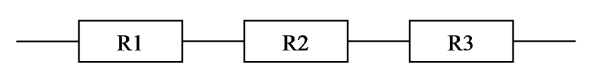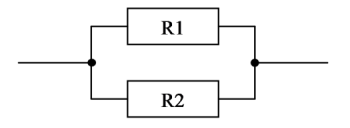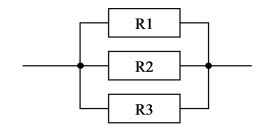# Resistor Series Parallel Formula

## To calculate the total resistance of the series circuit$$\begin{array}{l}R_{t} = R_{1}+R_{2}+R_{3}\end{array}$$

Where,

Rt= Total Resistance

R1 = Resistance 1

R2 = Resistance 2

R3 = Resistance 3

## Total Resistance Of 2 Resistors Connected parallel$$\begin{array}{l}R_{t} = \frac{R_{1}R_{2}}{R_{1}+R_{2}}\end{array}$$

Where,

Rt= Total Resistance

R1 = Resistance 1

R2 = Resistance 2

## Total Resistance Of 3 or more Resistors Connected parallel$$\begin{array}{l}\frac{1}{R_{t}}=\frac{1}{R_{1}}+\frac{1}{R_{2}}+\frac{1}{R_{3}}+——+\frac{1}{R_{n}}\end{array}$$

Where,

Rt= Total Resistance

R1 = Resistance 1

R2 = Resistance 2

R3 = Resistance 3

To know more about series and parallel connections with problems solved refer BYJU’S!!